Transcripted Summary

This is it. This is the section that makes this entire course worthwhile, because this is the coolest thing that you are going to learn how to do... I love doing this, this is the best thing…

What we're going to do is we're going to learn how to write code using `setInterval`, so it's going to keep going, that actually interacts with the application.

And you use the app as though it were a user — we're going to write an autonomous bot to interact with the user.

You can almost imagine, if we were doing multi-user testing, we could have a bot running in one browser doing things, deleting things, creating new to-dos. And we'd be over here doing our thing and we'll see the interaction with a normal user.

We might expose timing issues, locking issues, that kind of thing.

Let me just clear the application down [`localStorage.clear()`].

There's a couple of things I need to learn before I start here.

We already know `setInterval`; that would be useful. We already know how to create object; that will be useful.

What we don't know if how to randomly select things. Because I'm going to want to have something randomly create a todo. Randomly delete the todo. Randomly choose to click it.

So, I'm going to need some sort of random function in here.

Now JavaScript does have a random built-in, in the math library if I say — `Math.random()` — I will get a random number between 0 and 1.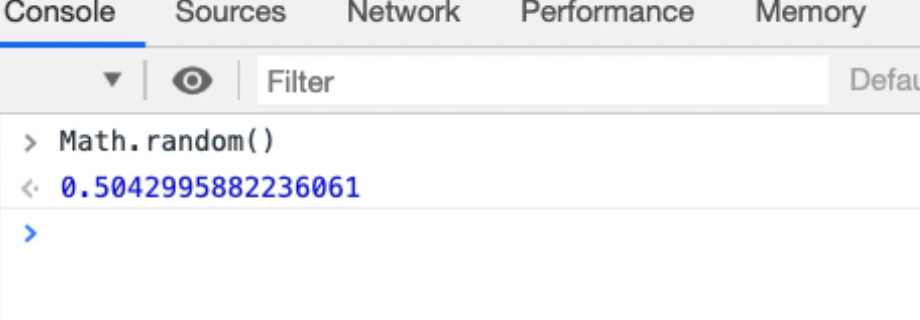I essentially just want integers, because I'm just going to say, “choose one of these particular 10 actions”, so I'll do that with a `floor` command.

If I say — `Math.floor(Math.random())` — at this point I'm not going to get a random number anymore because it's always going to `floor` it down to the nearest integer, which is always going to be 0.

So, I'm going to use a technique to say, “I want to convert this into a random number between 1 and 10, or 0 and 10”.

``````Math.floor(Math.random()*10)
``````

This will now give me a number between 0 and 10, not including 10.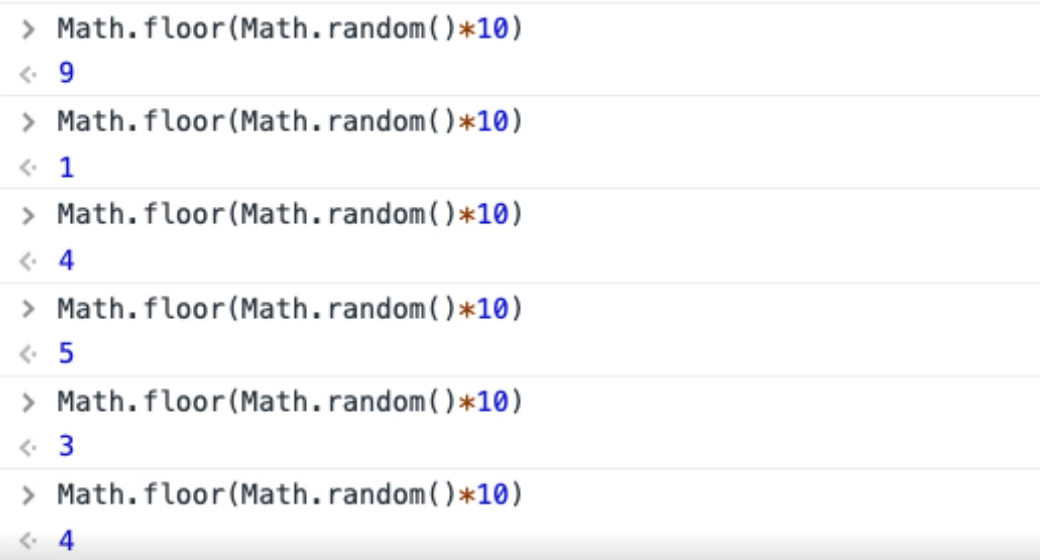So, 0 to 9, 10 numbers.

And now, if I had 10 functions I wanted to randomly select from, this would randomly give me an integer and I could do them.

How then do we find all the functions that we have access to?

Let me just set up the `autoTodo` object, which we’ve still got in snippets.

So, there's my vanillas todo objects [vanilla objects folder]. Here's my `autoTodo` object. I'm just going to run this snippet to set that up.

Now in `autoTodo` I’ve got that object, so I can now `autoTodo.toggleAll`.

There we go.

If I had a way of finding out what functions `autoTodo` is, I could randomly choose them if they were all in an array.

Fortunately, JavaScript has that functionality out of the box.

I'm going to create a `for` loop. I'm going to say,

``````for(var prop in autoTodo){
if(typeof autoTodo[prop] == 'function'){console.log(prop)}
}
``````

So, this loop is just going to say, “if the type of ‘autoTodo prop’ is a function, then I want to write that to the console.

I'll just run this then I'll explain it properly.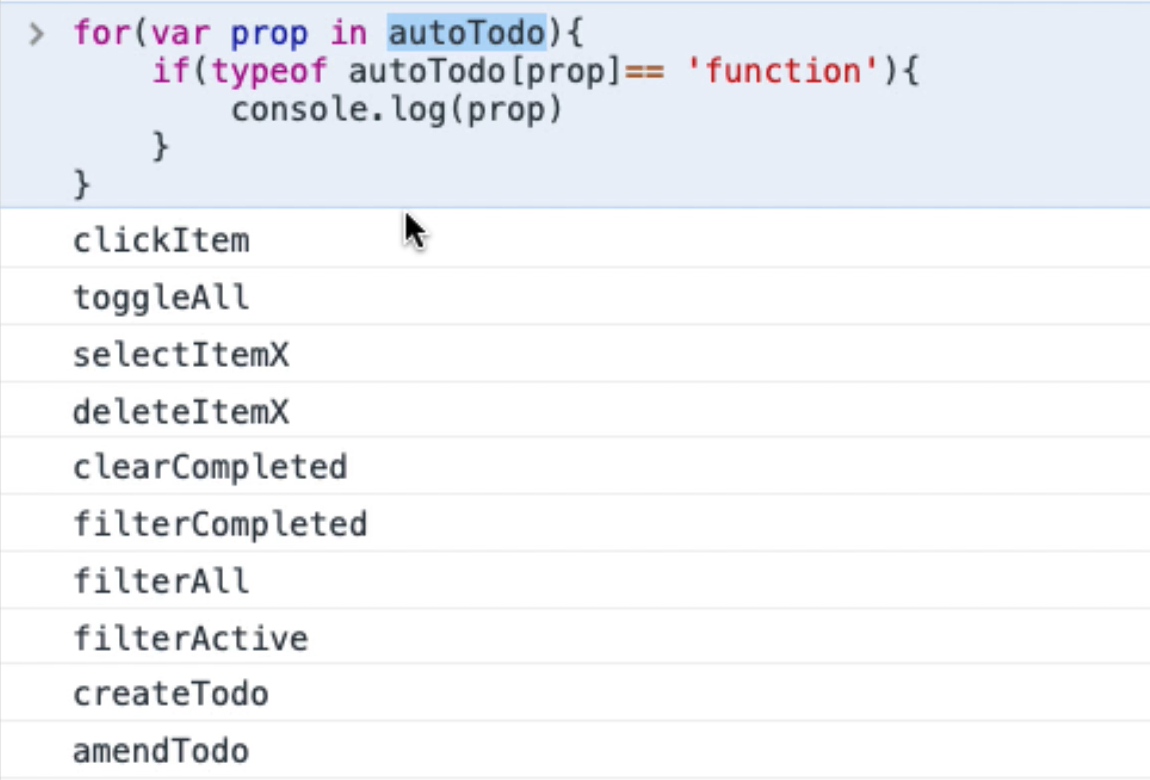There you go, there are all the functions that we wrote on `autoTodo`.

So, what does this do?

Well, every object in JavaScript is a function. A function is also essentially an array.

Here I'm saying, get me all the names of things, all the properties on `autoTodo` — this is using array indexing.

So, this is essentially a name or a value for the function. If that name or value is a function, then write out the name here. Again, this is one of [those things where] you just have to learn patterns.

Let me quickly show you arrays in JavaScript.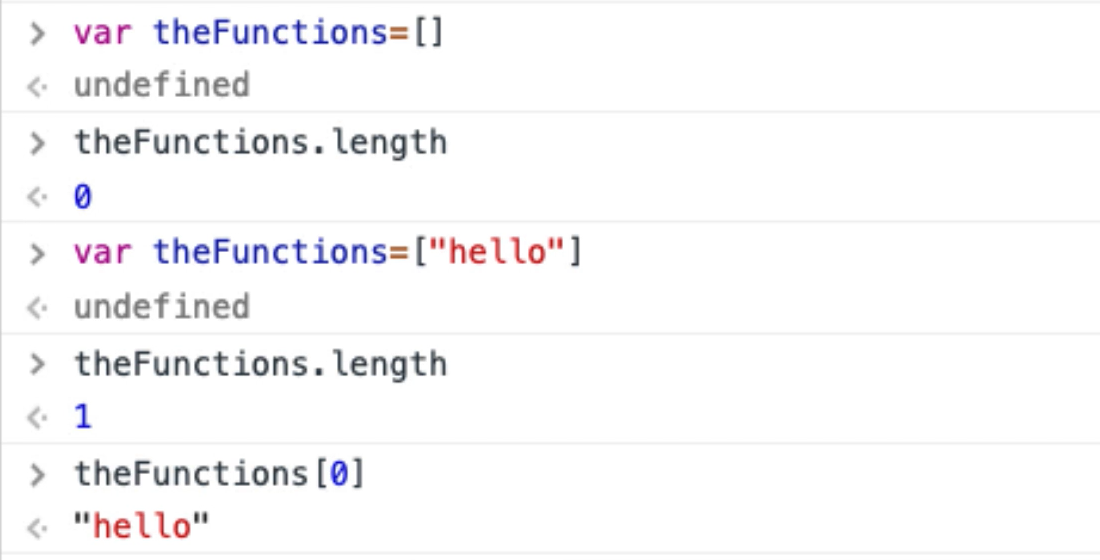If I say — `var theFunctions = []` — make this an empty array, let them call it “theFunctions”.

• So, `theFunctions` is an array, so it has a length — `theFunctions.length` — it has a length of 0.

• I could say — `var theFunctions = []` — now `theFunctions` has a length of 1, because there's 1 thing in it.

• At position 0 in that array — `theFunctions` — there is the String, “hello”.

• I can add things to it — `theFunctions.push(“there”)` — now there should be 2 things in that array [`theFunctions.length`]. Yup.

• The second thing, which is index 1 — `theFunctions` — should be “there”.

And, remember at the Console, I could just write `theFunctions` and I'll see the entire object listed.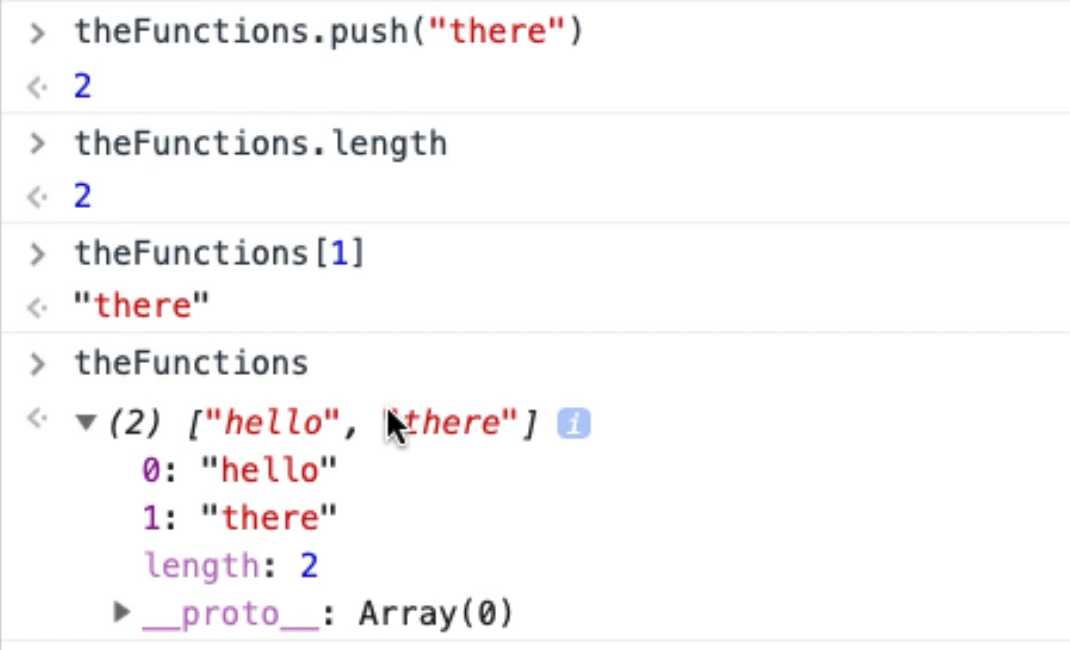JavaScript is a little bit of a strange language because every time we actually create an object, which is actually a function, it can also work as an array and all these public items are the index points within the array.

What is returned from that index point is the actual function itself.

Very simple, but we don't really have to get it completely clear in our head. It takes a little bit of time with JavaScript to get this in your head.

All we have to recognize is that there's a pattern where we can actually iterate over all of the functions and use them. So that's going to help us.

Now we actually have all the information that we need in order to write this, there's just one area of complexity we have to solve.

In the `autoTodo` object you can see that some of these functions take parameters. So, if I iterate over the functions, I have to know whether they take parameters or not.

I'm going to make this easy for myself, and what I'm actually going to do is create an object that wraps `autoTodo` around a random object that wraps `autoTodo`. So I don't have to worry about the parameters. It will handle that for me, so I'll have a kind of random `autoTodo` that knows when I'm selecting an item that it needs to identify a particular item and select that.

Let's start working on that.

I'm going to create a new snippet for this. I'm going to call it “random todo bot”, let's call it that.

This is going to be a function, I'll call it “rando”, and it's just going to be a new function, so an object.

``````var rando = new function(){

}
``````

And I'm just going to have private functions to handle the random generation, and it's going to call the `autoTodo` object to do its work.

First of all, I better have a private function for generating a random number, because I'm going to use that a lot. I will need to say what the upper level limit is, so then we've already seen this.

``````function getRandomInt(x){
return Math.floor(Math.random() * x);
}
``````

So, if I say — `getRandomInt(10)` — I will get a random number between, or including, 0 to 9.

I will also need the ability to choose a random item in this list.

How do I get these items on this list?

Let me jump back to the Console.

So, if I say `document.`...

And do you remember who we get all the elements off the screen?

We use `querySelectorAll`. Then I will need to write a CSS query to access the todo items.

If I quickly Inspect this.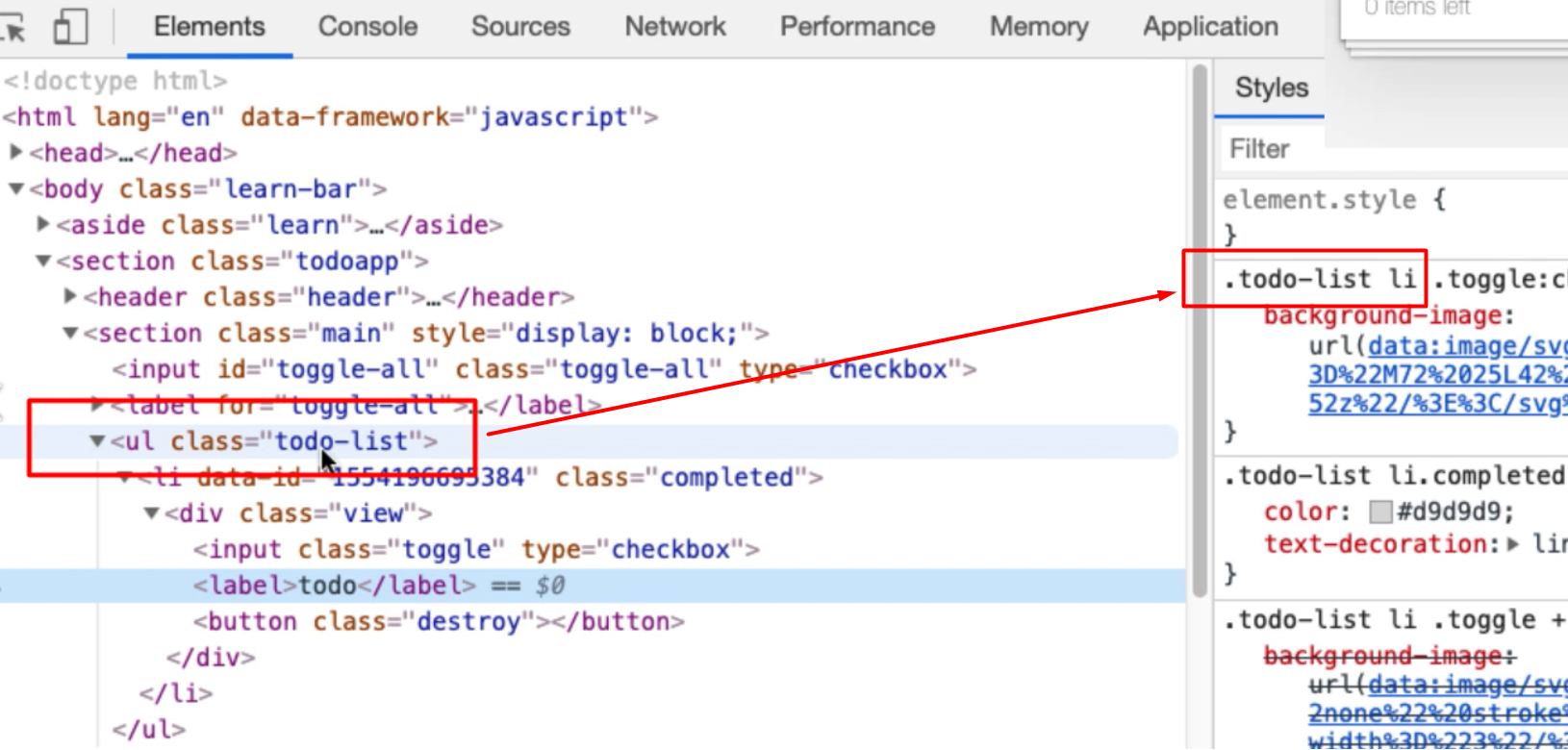And you can see it's building this up as we go. There's our `NodeList`.

And that looks like it's a collection which means I can say, give me the length.

So, there's 1 item there, so I know now how to get the number of things in there.

Let me just double check, “todo again”. Run this, there should be 2.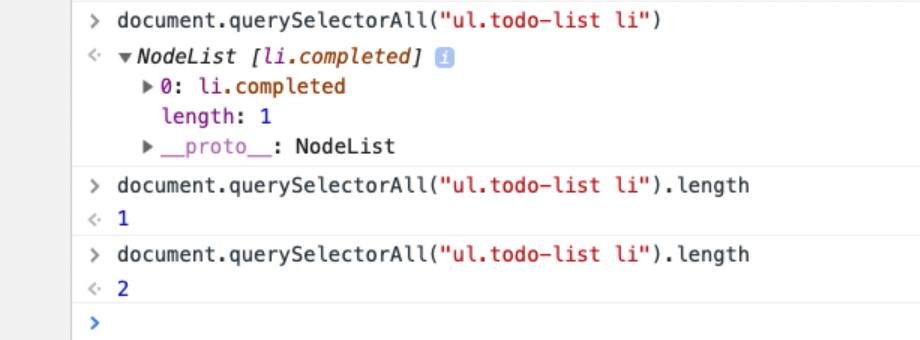There we go.

Given we have this number of items in the list, and I only want to try and choose one if there are items there.

• If `max` if equals 0, then I will say there are no items. And then I will just return 0. So, anyone calling this function, if they get 0 back it means don't do anything; there's nothing to do.

• So, if there are items, then I'll want to say — `x = getRandomInt`— up to this max.

I will want to use this in CSS queries. And CSS queries are 1 index.

``````function getRandomItemIndex(){
max = document.querySelectorAll("ul.todo-list li").length;
if(max===0){
console.log("no items to choose from");
return 0;
}
x = getRandomInt(max)+1;
return x;
}
``````

So, this is a private function that lets me choose an item, or find an item number index, from here.

Let's just quickly use that.

In the `autoTodo` we've got the `selectItemX`.

What I'm going to do, I'm going to say — `this.selectItemX` — which is a function, and none of my functions in random are going to take any parameters because we're going to randomly generate them.

• I'm going to say —`x = getRandomItemIndex` — because I want to pick one in the list.

• And, if X is greater than 0, because I only want to do it if there's something in the list.

• Then I want to see a `console.log` because this will help me understand what the bot is doing.

``````this.selectRandomItem = function () {
x = getRandomItemIndex();
if(x>0){
console.log("select item " + x);
autoTodo.selectItemX(x);
}
}
``````

Let me try and run this.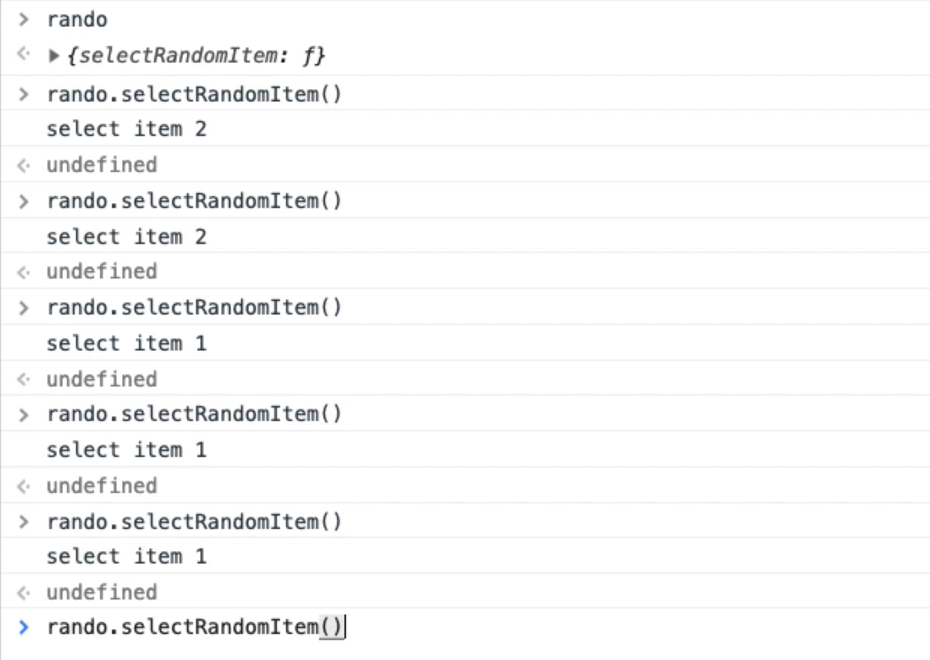That [`rando`] would just create the random object.

There we go — `rando.SelectRandomItem()`has been exposed as a public function.

Now I have something that is randomly choosing an item from the list and selecting it. And my `rando` object is just in charge of creating the random data that is going to pass onto the `autoTodo` object that actually does the work.

So, I have multiple layers of abstraction here.

I've got the `autoTodo` object, which is an object that we've been using to help us do the work for automating it.

And I'm creating an abstraction there on top of that, so that I don't have to worry about creating the random data that is passed through to that, and I can just select it.

Now it's very easy for me to create a wrapper for `toggleAll`, because it's simply going to be `this.toggleAll`, which is a function. And that's just going to `console.log`.

``````this.toggleAll = Toggle All");
console.log("toggle all");
autoTodo.toggleAll();
}
``````

And let's just check that works in the Console`rando.toggleAll` —there we go.

So, any function that doesn't take a parameter is very easy for me to wrap.

Let me just check the vanilla objects again, because I'm just building up the patterns, then I'm going to go away and write the code, so I don't have to slow that down for you.

We will finish creating the bot in part 2 of this chapter.

## Resources

Quiz

The quiz for this chapter can be found in section 12.3